+关注继续查看

# 2. 算术操作符

+   -   *    /   %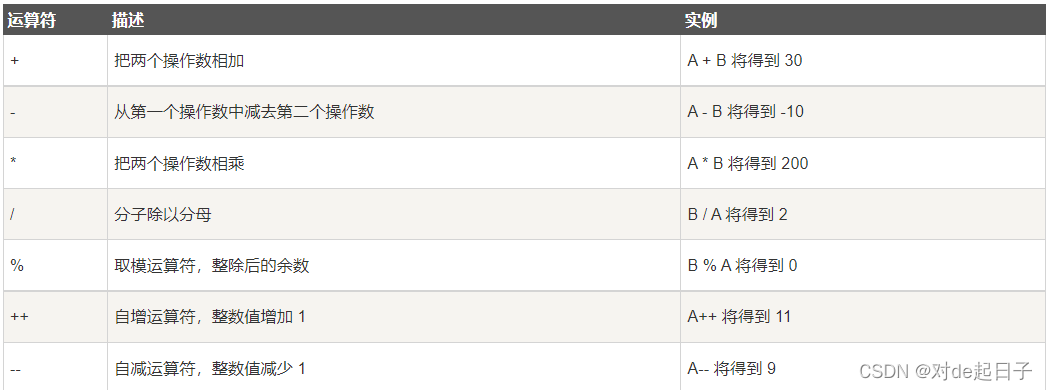1. 除了 % 操作符之外，其他的几个操作符可以作用于整数和浮点数。
2. 对于 / 操作符如果两个操作数都为整数，执行整数除法。而只要有浮点数执行的就是浮点数除法。
3. % 操作符的两个操作数必须为整数。返回的是整除之后的余数。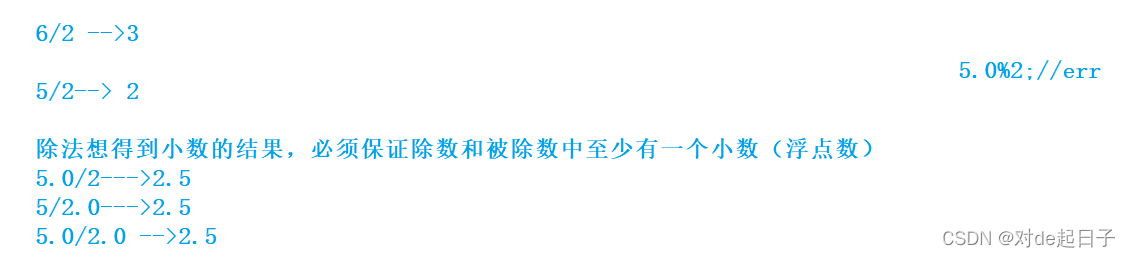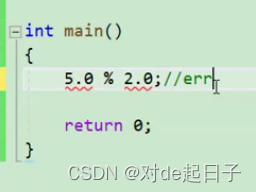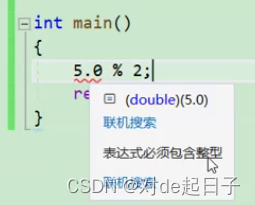# 3. 移位操作符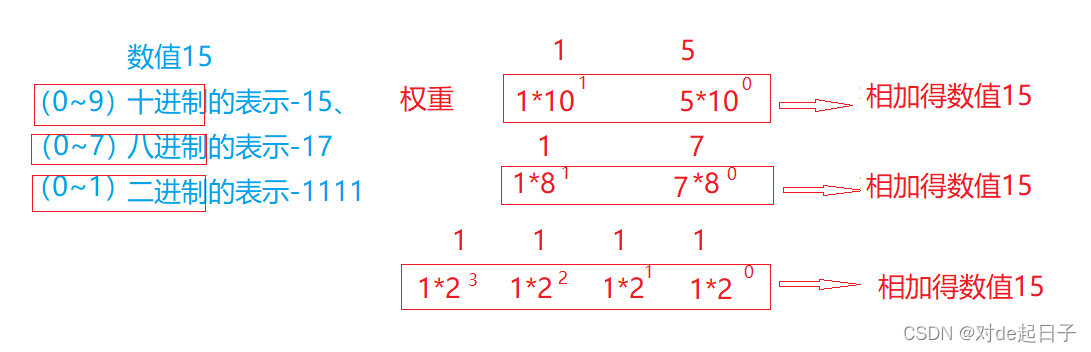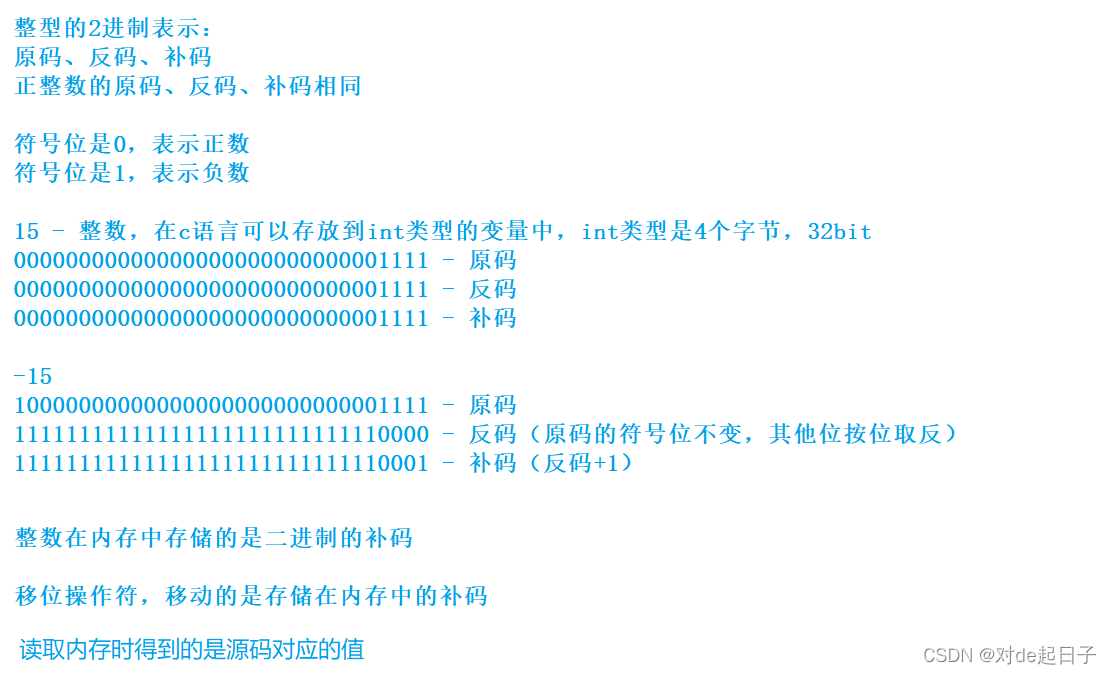## 3.1 左移操作符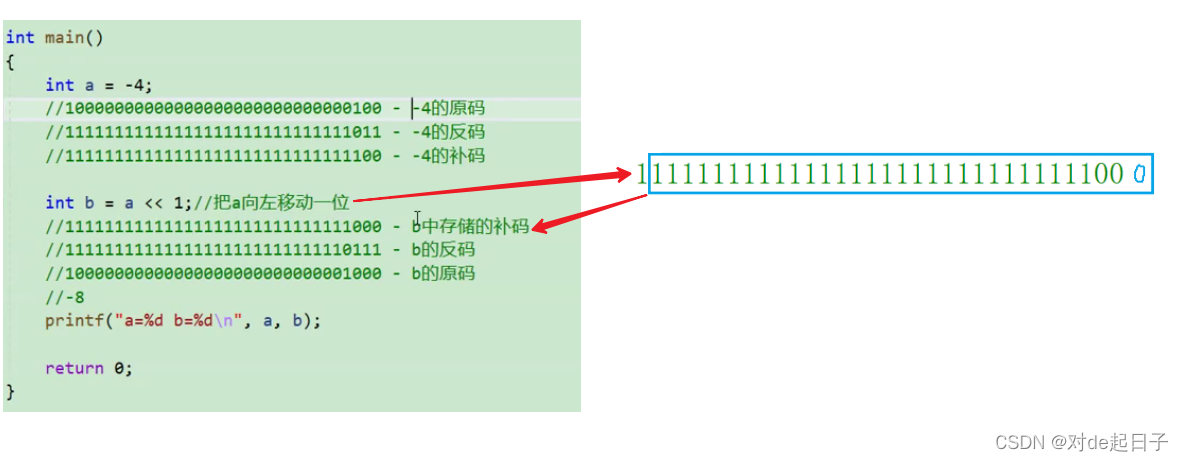## 3.2 右移操作符

1. 逻辑移位
左边用0填充，右边丢弃
2. 算术移位
左边用原该值的符号位填充，右边丢弃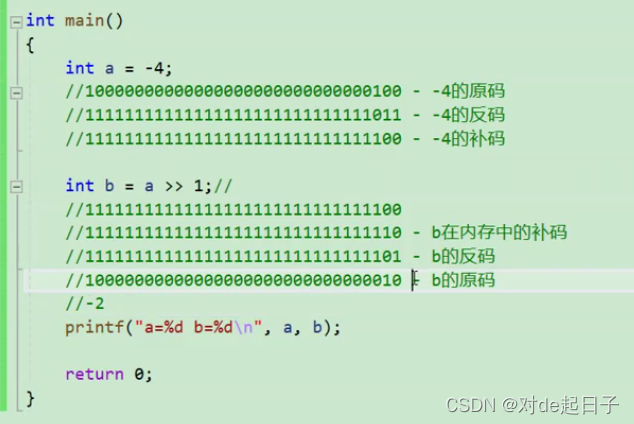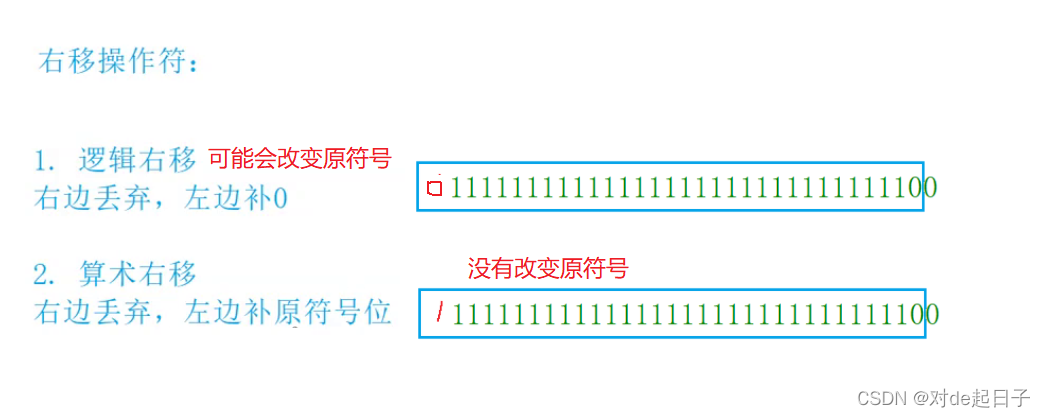int num = 10;
num>>-1;//error

# 4. 位操作符

2.位操作符作用于二进制数列

 位操作符 名称 作用 & 按位与 有0则0，同1则1 | 按位或 有1则1 ^ 按位异或 相同为0，相异为1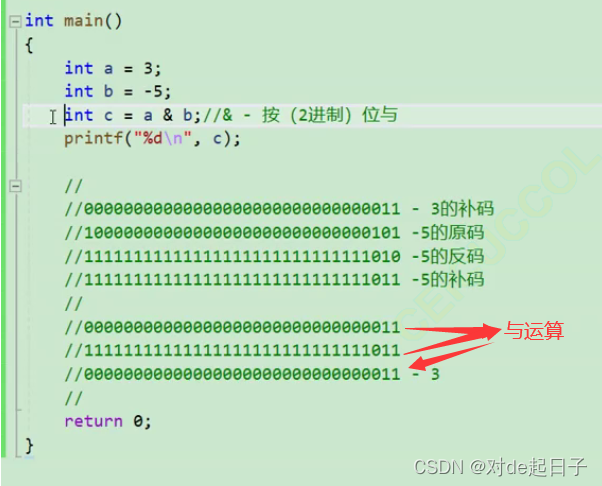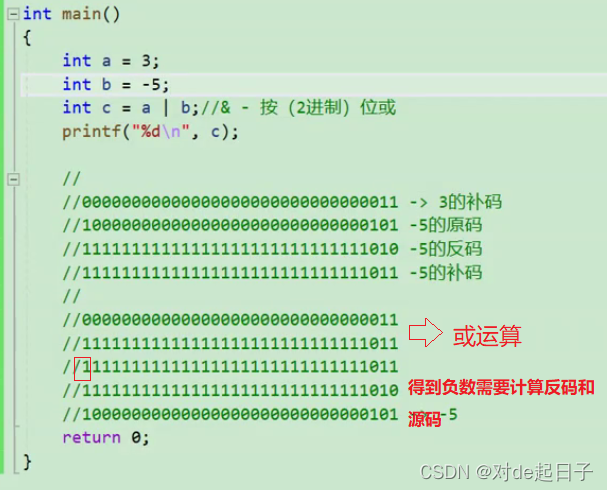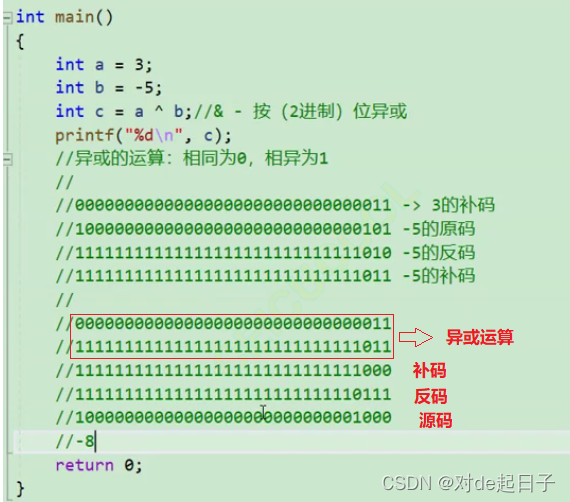• 一道变态的面试题：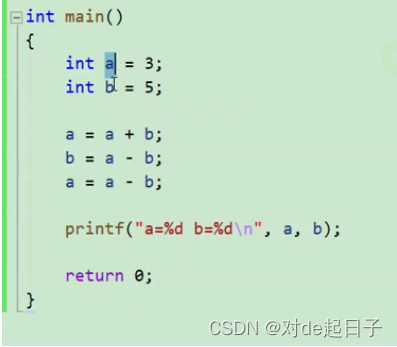1.相同为0，0与任何数异或结果都为这个数

2.异或支持交换律

//3^3 = 0
//5^5=0
//3^5=6
//3^5^5=3
//3^5^3=5
//
//000
//011
//011
//011
//101
//110
//a^a=0
//0^a=a
//异或是支持交换律
//3^5^3
//3^3^5
//
int main()
{
int a = 3;
int b = 5;
a = a ^ b;
b = a ^ b;//b=a ^ b ^ b
a = a ^ b;//a^b^a
//a = a + b;
//b = a - b;
//a = a - b;
printf("a=%d b=%d\n", a, b);
return 0;
}

//方法1

#include <stdio.h>
int count_bit( int m)
{
int count = 0;
while (m)
{
if (m % 2 == 1)
count++;
m /= 2;
}
return count;
}
int main() {
int n = 0;
scanf("%d", &n);
int num = count_bit(n);
printf("%d\n", num);
return 0;
}

int count_bit(unsigned int m)

//方法2：
#include <stdio.h>
int count_bit(n)
{
int i = 0;
int count = 0;
for (i = 0; i < 32; i++)
{
if (1 == ((n >> i) & 1))
count++;
}
return count;
}
int main()
{
int n = 0;
scanf("%d", &n);
int num = count_bit(n);
printf("%d\n", num);
return 0;
}

//方法3：

int count_bit(int m)
{
int count = 0;
while (m)
{
m = m & (m - 1);
count++;
}
return count;
}
int main() {
int n = 0;
scanf("%d", &n);
int num = count_bit(n);
printf("%d\n", num);
return 0;
}
//n=11
//n = n&(n-1)
//1011 - n
//1010 - n-1
//1010 - n
//1001 - n-1
//1000 - n
//0111 - n-1
//0000 - n
//根据上述规律可知，有几个n&(n-1)就有多少个1

# 5. 赋值操作符

int weight = 120;//体重
weight = 89;//不满意就赋值
double salary = 10000.0;
salary = 20000.0;//使用赋值操作符赋值。

int a = 10;
int x = 0;
int y = 20; a = x = y+1;//连续赋值

x = y+1; a = x;

# 复合赋值符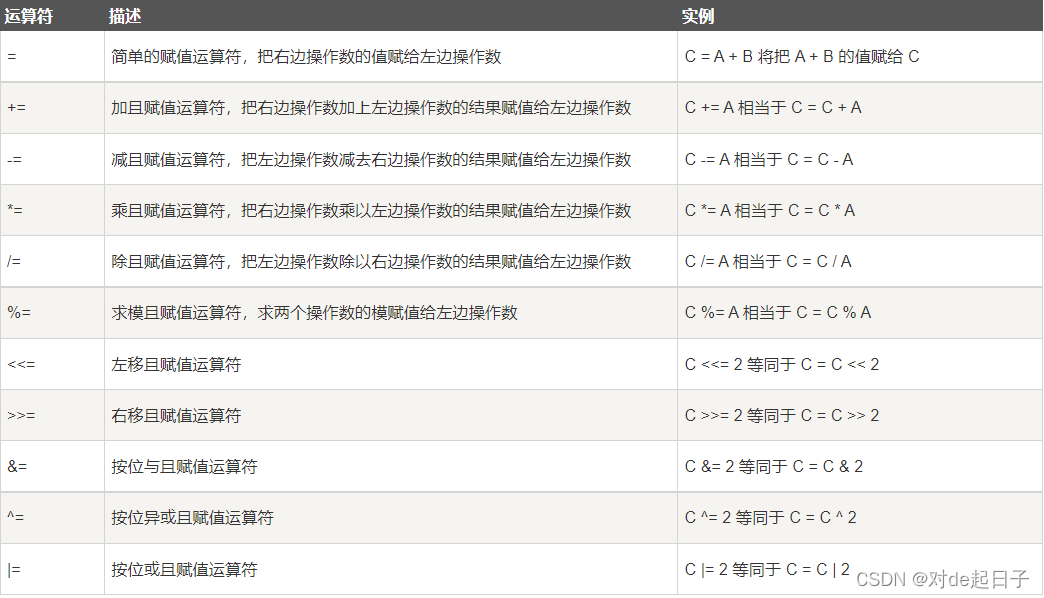int x = 10;
x = x+10;
x += 10;//复合赋值
//其他运算符一样的道理。这样写更加简洁。

# 6. 单目操作符

## 6.1 单目操作符介绍

! 逻辑反操作

- 负值

+ 正值

& 取地址

sizeof 操作数的类型长度（以字节为单位）

~ 对一个数的二进制按位取反

– 前置、后置–

++ 前置、后置++

* 间接访问操作符(解引用操作符) (类型) 强制类型转换

• ！举例
int main()
{
int flag = 0;
//if (!flag)
if(flag == 0)
{
printf("hehe\n");
}
return 0;
}
• &和解引用操作符的举例
int main()
{
int a = 10;
char c = 0;
//  printf("%p\n", &a);//取出地是二进制数列
//  printf("%p\n", &c);
int* pa = &a;//& - 取地址操作符  pa是指针变量  int*是类型
//*pa = 20;//* - 解引用操作符    把a拿出来进行赋值
*pa = 20;
return 0;
}
• sizeof操作符举例
int main()
{
short s = 10;
int a = 2;
s = a + 5;
printf("%zu\n", sizeof(s = a + 5));//输出结果为2，sizeof（）内部放的表达式不会计算
printf("%d\n", s);//，输出结果为10
int a = 10;
int* p;
int arr;
printf("%zu\n", sizeof(a));//int  4
printf("%zu\n", sizeof a);//int  4
printf("%zu\n", sizeof(int));//int  4
//printf("%zu\n", sizeof int);//err
printf("%zu\n", sizeof(p));//int* 4
printf("%zu\n", sizeof(arr));//int  40
printf("%zu\n", sizeof(arr));//int 4
return 0;
}

⭐️ 这里需要注意的是sizeof（数组名）是指数组整体的类型长度，而不是数组首元素的类型长度，sizeof（）内部放的表达式不会计算。

• 原理：
在文件转向可执行程序的时候在编译阶段，编译器就进行了判断，进行了截断把后面括号中两个字节的内容给了short类型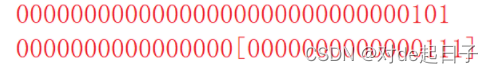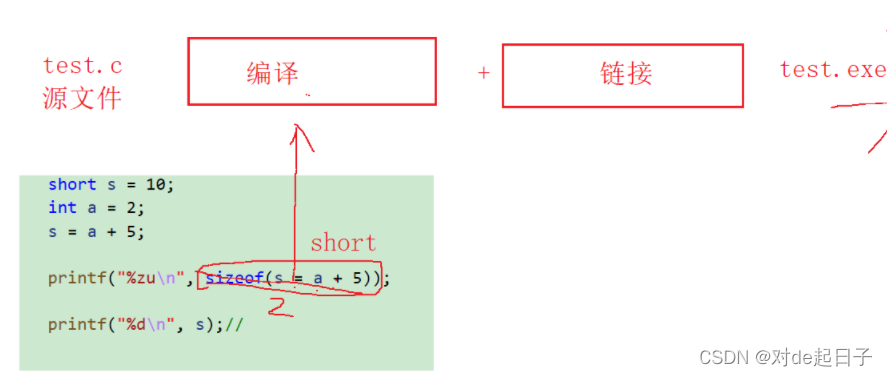• ~按位取反操作符
• 代码与原理展示：
int main()
{
int a = 0;
/*00000000000000000000000000000000 - ~按位取反
11111111111111111111111111111111 - 内存中-补码
11111111111111111111111111111110 -反码（补码减1）
10000000000000000000000000000001 -源码（反码符号位不变其他位按位取反）

-1*/
printf("%d\n", ~a);
return 0;
}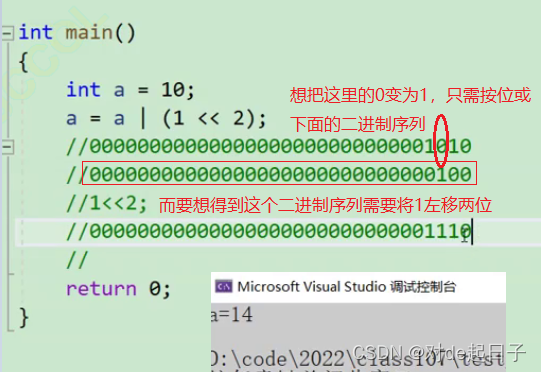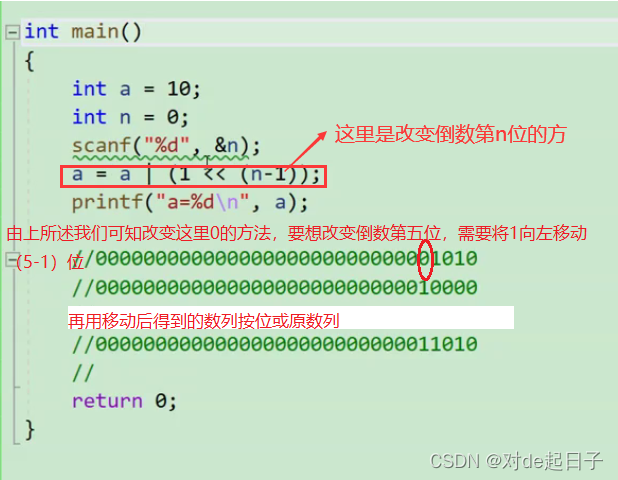int main()
{
int a = 10;
int n = 0;
scanf("%d", &n);
//把a的第n位置为1
a = a | (1 << (n-1));
printf("a=%d\n", a);
//把a的第n位置为0
a = a & ~(1 << (n - 1));
printf("a=%d\n", a);
//00000000000000000000000000001010
//00000000000000000000000000010000
//1<<2;
//00000000000000000000000000011010
//11111111111111111111111111101111
//00000000000000000000000000010000
//00000000000000000000000000001010
return 0;
}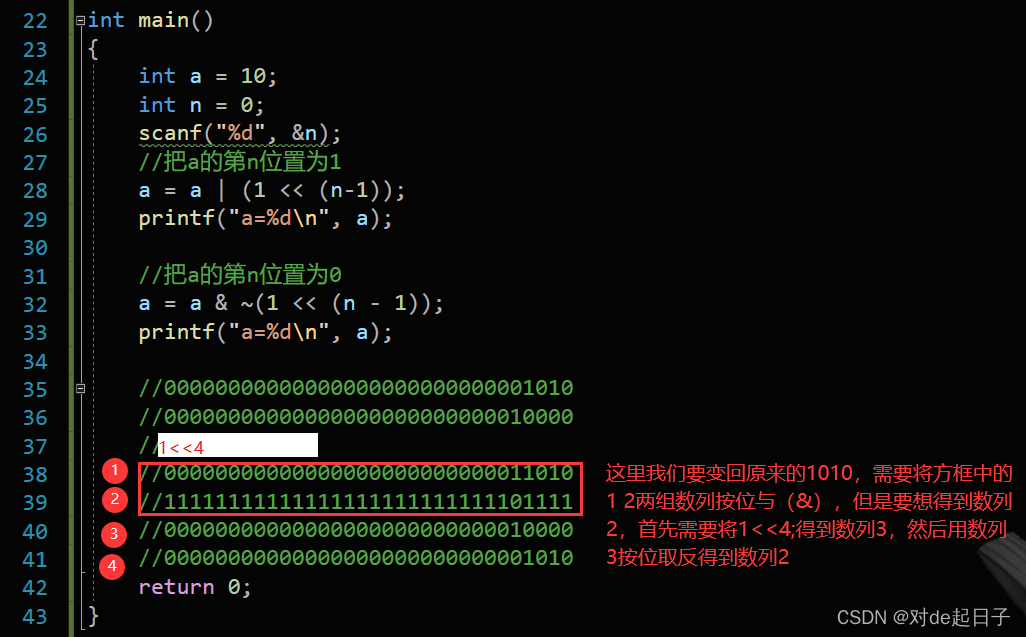## 6.2 sizeof 和 数组

#include <stdio.h>
void test1(int arr[]) {
printf("%d\n", sizeof(arr));//(结果4)
}
void test2(char ch[]) {
printf("%d\n", sizeof(ch));//(结果4)
}
int main() {
int arr = { 0 };
char ch = { 0 };
printf("%d\n", sizeof(arr));//(结果40)
printf("%d\n", sizeof(ch));//(结果10)
test1(arr);
test2(ch);
return 0;
}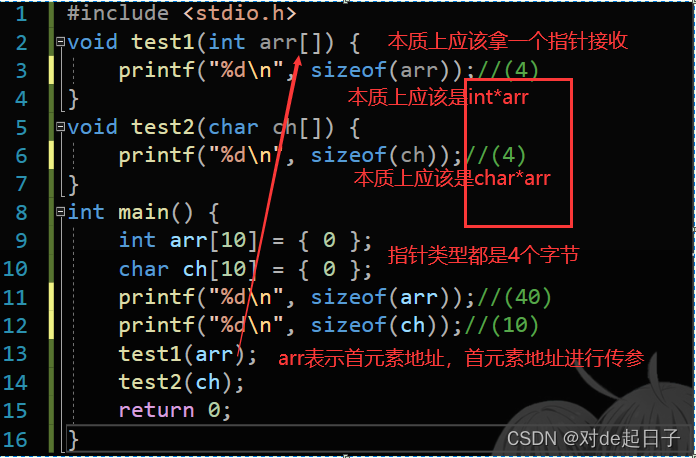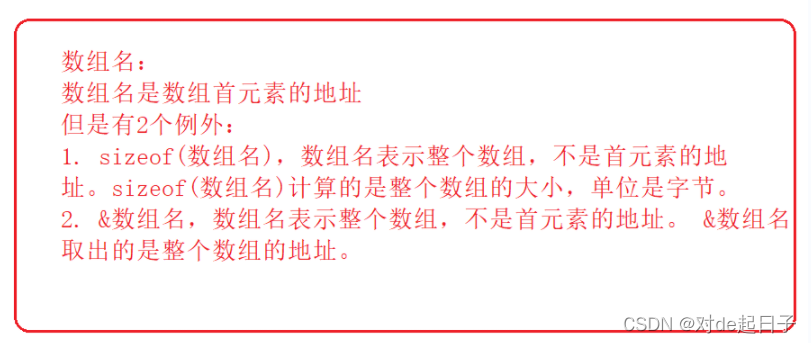• ++ - -运算符
关于++和–运算符，前面的文章中提到过，但下面的图片也是一目了然
若图片看不太懂，还有代码供参考😏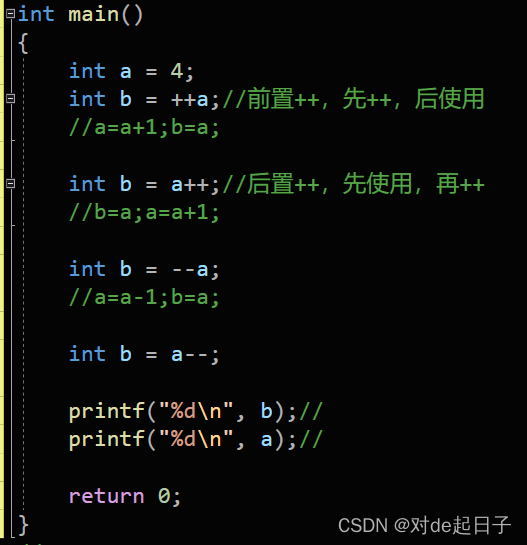//++和--运算符
//前置++和--
#include <stdio.h>
int main()
{
int a = 10;
int x = ++a;
//先对a进行自增，然后对使用a，也就是表达式的值是a自增之后的值。x为11。
int y = --a;
//先对a进行自减，然后对使用a，也就是表达式的值是a自减之后的值。y为10;
return 0; }
//后置++和--
#include <stdio.h>
int main()
{
int a = 10;
int x = a++;
//先对a先使用，再增加，这样x的值是10；之后a变成11；
int y = a--;
//先对a先使用，再自减，这样y的值是11；之后a变成10；
return 0; }
• 强制类型转换
如图，当我们将一个小数想要赋值给int型的变量时，编译器会报错，这里就需要用到强制类型转换，下面的代码中我们就运用了强制类型转换，只要认真观察区别，就可以学会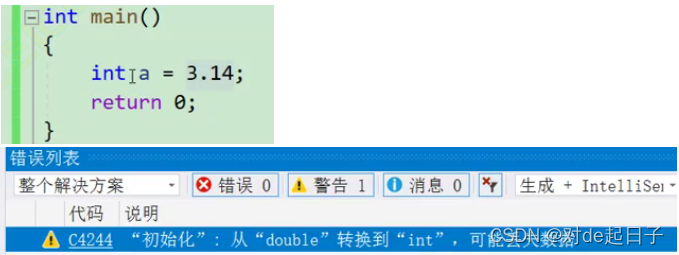int main()
{
int a = (int)3.14;
printf("%d\n", a);
return 0;
}

# 7. 关系操作符

>

>=

<

<=

!= 用于测试“不相等”

== 用于测试“相等”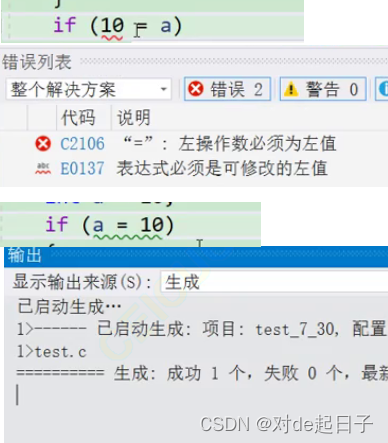int main()

{

char arr[] = “abcdef”;

==不能比较2个字符串的内容，实际上比较的是2个字符串的首字符的地址,所以这样写是错误的

if (arr == “abcdef”)

{

printf(“==\n”);

}

return 0;

}

# 8. 逻辑操作符

&& 逻辑与

|| 逻辑或

1&2----->0

1&&2---->1

1|2----->3

1||2---->1

“&&” 同理，若左边的操作数为0，则就不继续执行其右边的操作数

int main()
{
int a = 3;
int b = 0;
if (a && b)  //这里的&&仅仅判断真假，如果两边都是真，则继续执行，非0即为真
//if (a || b) //同样的，有真则为真
{
printf("呵呵\n");
}
return 0 ;
}
int main()
{
int age = 20;
18~36 青年
if (age >= 18 && age <= 36)
{
printf("青年\n");
}
int main()
{
int year = 2000;
if (((year % 4 == 0) && (year % 100 != 0)) || (year % 400 == 0))
printf("闰年");//这里的&&是并且的意思  ||是或的意思
return 0;
}

360笔试题

#include <stdio.h>
int main()
{
int i = 0,a=0,b=2,c =3,d=4;
i = a++ && ++b && d++;
//i = a++||++b||d++;
printf("a = %d\n b = %d\n c = %d\nd = %d\n", a, b, c, d);
return 0;
}

//程序输出的结果是什么？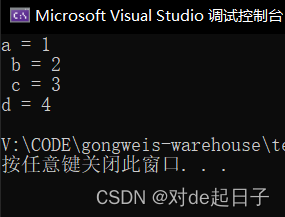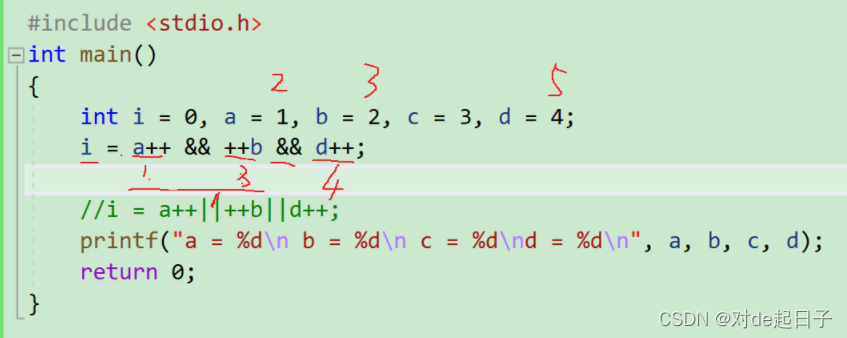输出结果为2335；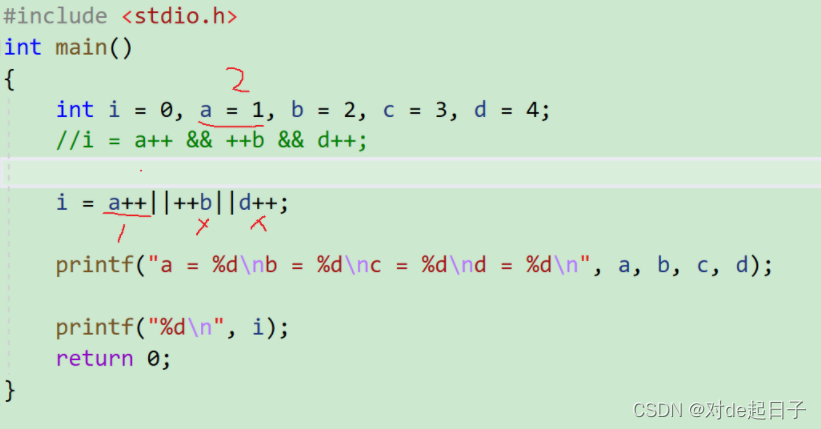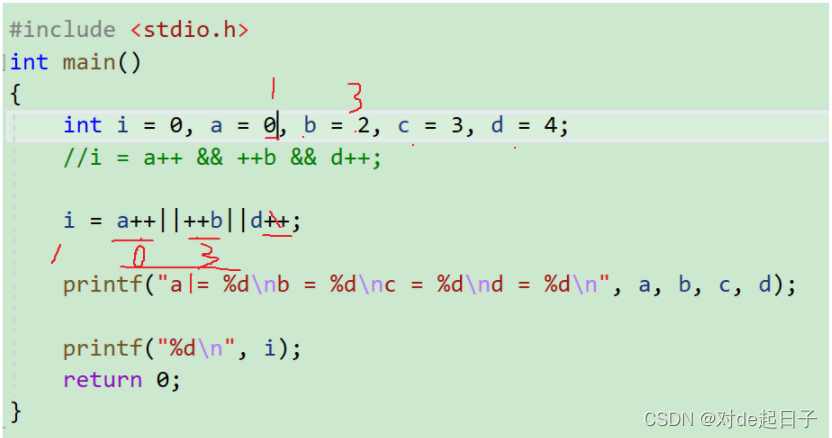# 9. 条件操作符

exp1 ? exp2 : exp3

int main()
{
int a = 3;
int b = 0;
if (a > 5)
b = 3;
else
b = -3;
return 0;
}

b = ((a > 5) ? 3 : -3);

2.使用条件表达式实现找两个数中较大值。

int m = (a > b ? a : b);

# 10. 逗号表达式

exp1, exp2, exp3, …expN

//代码1
int a = 1;
int b = 2;
int c = (a>b, a=b+10, a, b=a+1);//逗号表达式
c是多少？
13
//代码2
if (a =b + 1, c=a / 2, d > 0)
//代码3
a = get_val();
count_val(a);
while (a > 0)
{
//业务处理
a = get_val();
count_val(a);
}

while (a = get_val(), count_val(a), a>0)
{
//业务处理
}

# 11. 下标引用、函数调用和结构成员

• 1. [ ] 下标引用操作符
注意：[ ]不是定义数组时的操作符，而是在要访问数组的第某个元素时所使用的操作符
arr;访问数组的第5个元素，
这里的[ ]就交下标引用操作符，
操作数是arr和4
操作数：一个数组名 + 一个索引值
• 2. ( ) 函数调用操作符
接受一个或者多个操作数：第一个操作数是函数名，剩余的操作数就是传递给函数的参数。
void test(int x, int y)
{
}
void test2()
{}
int main()
{
test2();
//操作数：test2
test(3, 4);//()函数调用操作符
//操作数：test,3,4
return 0;
}
• 3. 访问一个结构的成员

. 结构体.成员名

-> 结构体指针->成员名

//结构体成员

//书：书名 + 定价

struct Book
{
char name;
int price;
};
int main() {
struct Book sb = { "C语言", 55 };
printf("%s %d\n", sb.name, sb.price);//结构体变量.结构体成员名
struct Book* ps = &sb;
printf("%s %d\n", (*ps).name, (*ps).price);//ps指向sb，*ps找到sb
printf("%s %d\n", ps->name, ps->price);//结构体指针->结构体成员名
//->name找到了ps所指向的对象sb的name
return 0;
}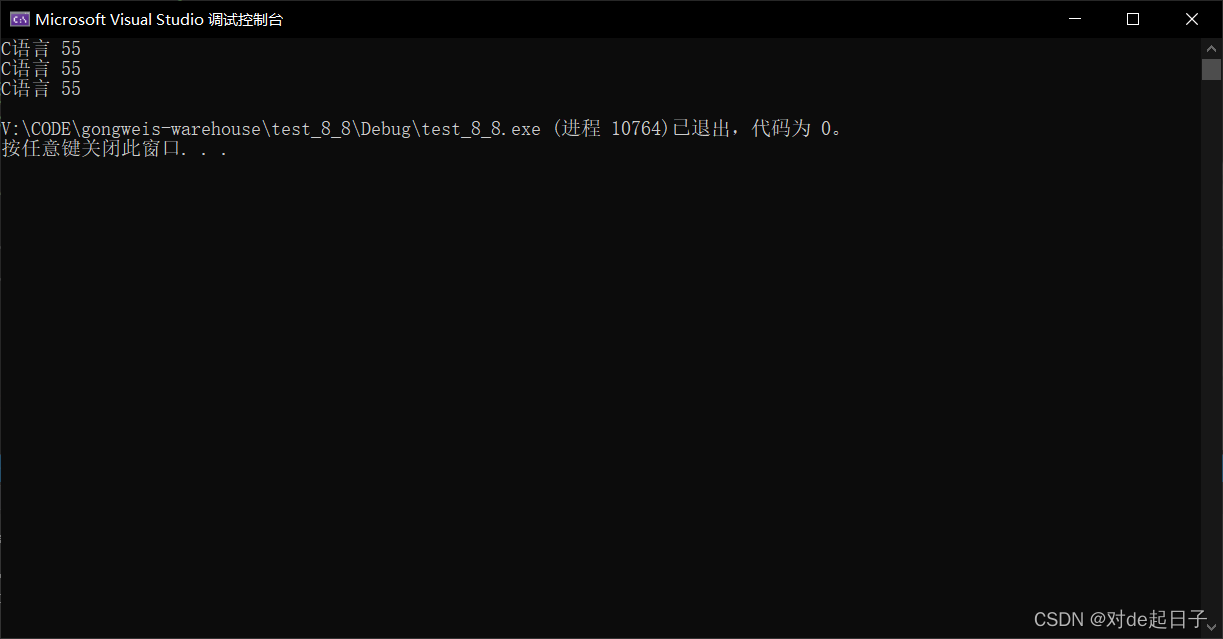# 12. 表达式求值

## 12.1 隐式类型转换

C的整型算术运算总是至少以缺省整型类型的精度来进行的。

//负数的整形提升
char c1 = -1;

1111111

11111111111111111111111111111111
//正数的整形提升
char c2 = 1;

00000001

00000000000000000000000000000001
//无符号整形提升，高位补0

//实例1
int main()
{
char a = 5;
char b = 126;
char c = a + b;
printf("%d\n", c);

return 0;
}

b和c的值被提升为普通整型，然后再执行加法运算。

⭐️例1:

int main()
{
char a = 5;//截断
char b = 126;//截断
char c = a + b;//截断
//00000000000000000000000000000101
//00000101 - a
//00000000000000000000000001111110
//01111110 - b
//整型提升
//00000000000000000000000000000101-a
//00000000000000000000000001111110-b
//00000000000000000000000010000011
//10000011 - c
printf("%d\n", c);
//%d 十进制的方式打印有符号整数
//11111111111111111111111110000011
//11111111111111111111111110000010
//10000000000000000000000001111101
//-125
//
return 0;
}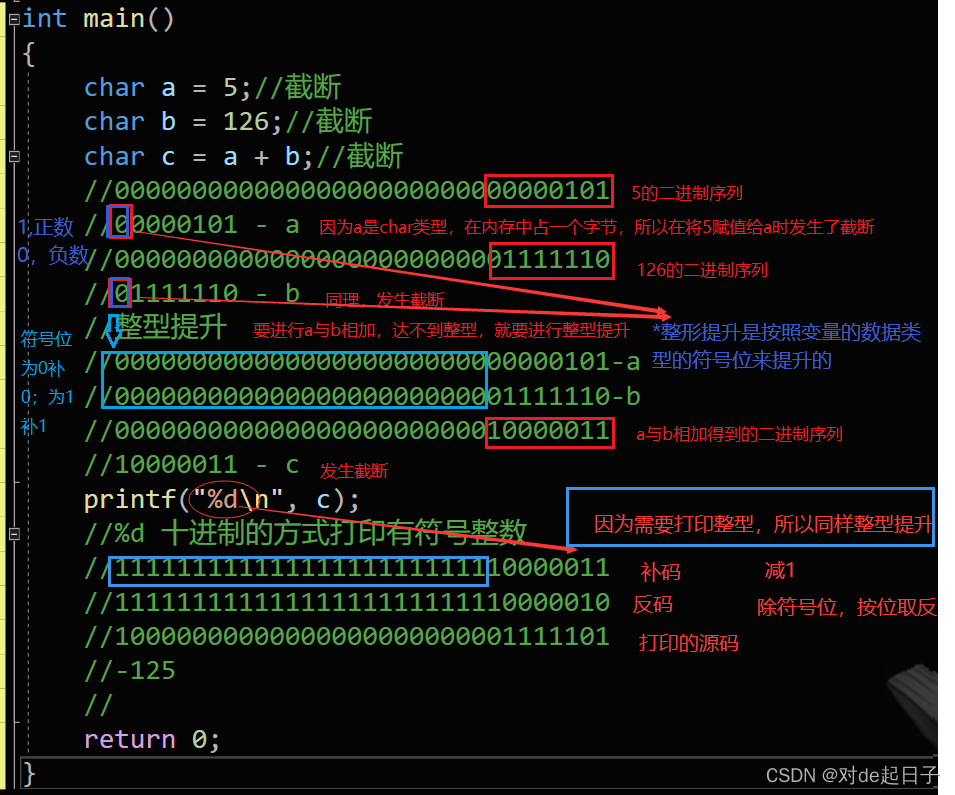⭐️例2:

int main()
{
char a = 0xb6;
//10110110
//
short b = 0xb600;
int c = 0xb6000000;
if (a == 0xb6)
printf("a");
if (b == 0xb600)
printf("b");
if (c == 0xb6000000)
printf("c");
return 0;
}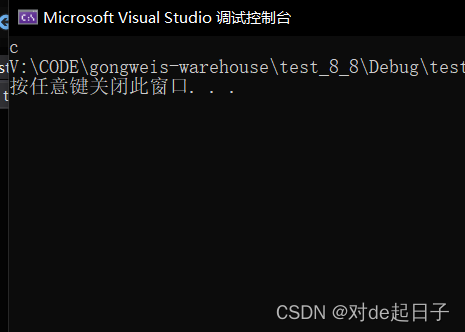a,b整形提升之后,变成了负数,所以表达式 a0xb6 , b0xb600 的结果是假,但是c不发生整形提升,则表

⭐️例3:

int main()
{
char c = 1;
printf("%u\n", sizeof(c));
printf("%u\n", sizeof(+c));
printf("%u\n", sizeof(-c));
return 0;
}

## 12.2 算术转换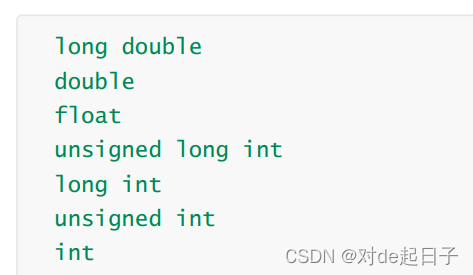float f = 3.14;

int num = f;//隐式转换，会有精度丢失

## 12.3 操作符的属性

1. 操作符的优先级
2. 操作符的结合性
3. 是否控制求值顺序。
两个相邻的操作符先执行哪个？取决于他们的优先级。如果两者的优先级相同，取决于他们的结合性。
操作符优先级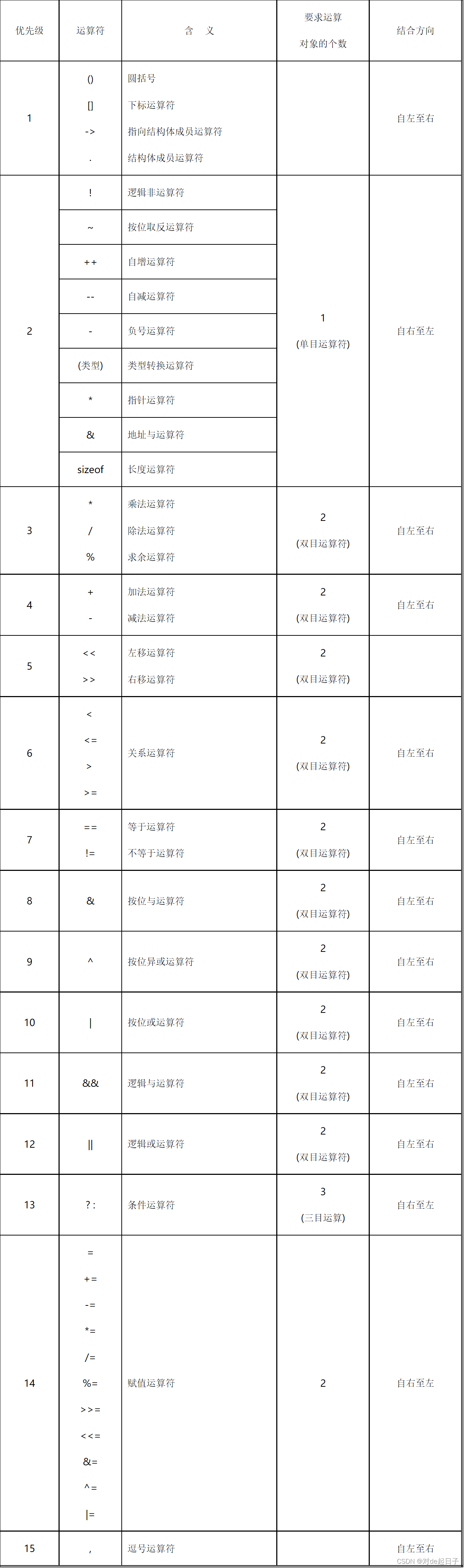//表达式的求值部分由操作符的优先级决定。

//表达式1

ab + cd + e*f

ab
c
d

ab + cd

ef
a
b + cd + ef

ab
c
d

ef
a
b + cd
a
b + cd + ef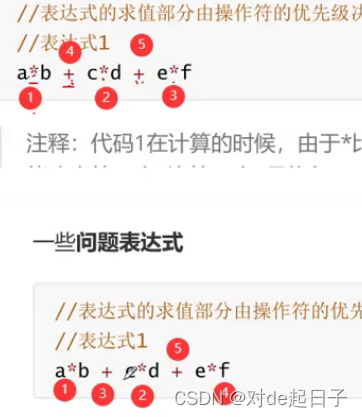//表达式2

c + --c;

//代码3-非法表达式

int main()

{

int i = 10;

i = i-- - --i * ( i = -3 ) * i++ + ++i;

printf(“i = %d\n”, i);

return 0;

}

//代码4
int fun()
{
static int count = 1;
return ++count;
}
int main()
{
answer = fun() - fun() * fun();
return 0;
}

//代码5
#include <stdio.h>
int main()
{
int i = 1;
int ret = (++i) + (++i) + (++i);
printf("%d\n", ret);
printf("%d\n", i);
return 0;
}

Linux环境的结果：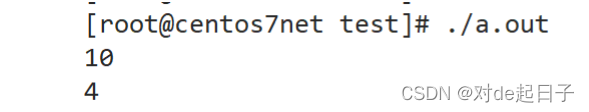VS2013环境的结果：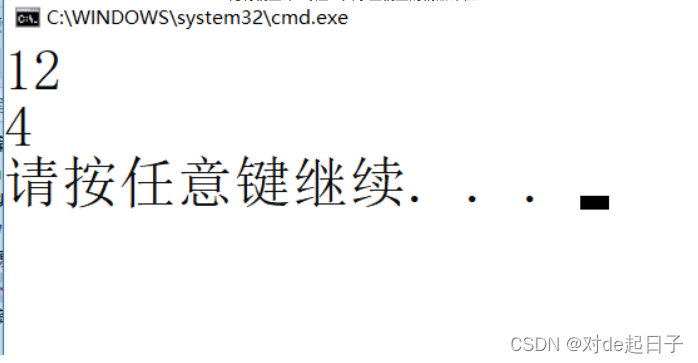0 0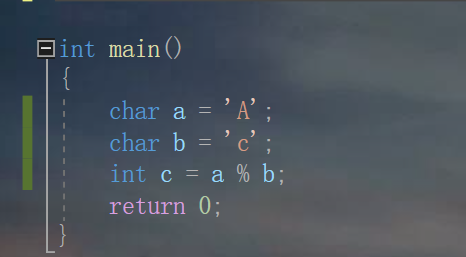0基础C语言保姆教程——第六节 操作符、表达式和语句

0 00 0C语言第二章 数据类型，运算符和表达式总结【完美补充文字版】（超级详细）
C语言第二章 数据类型，运算符和表达式总结【完美补充文字版】（超级详细）
0 0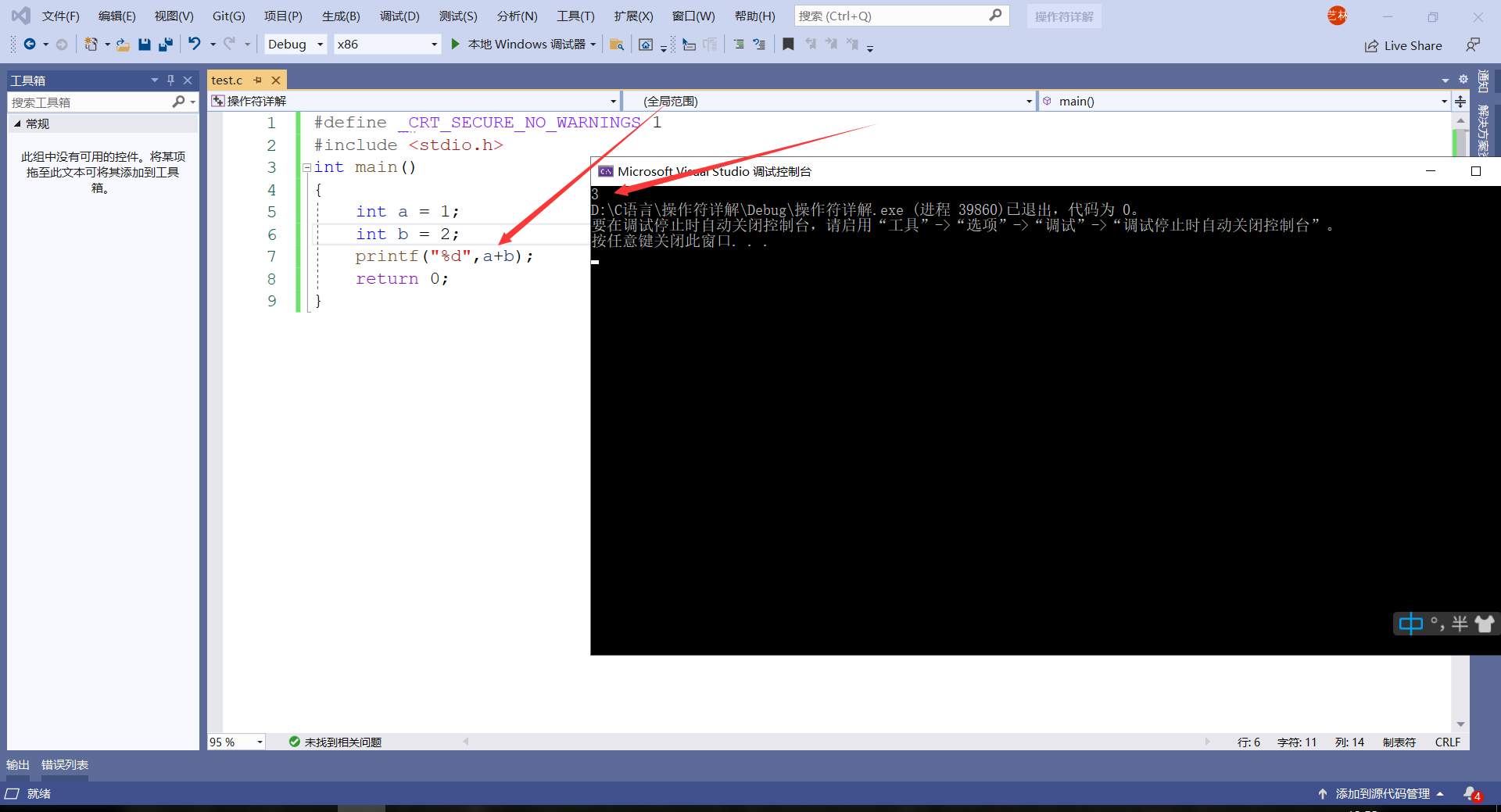【C语言】 操作符的困惑都在这里解决! (操作符详细解析)

0 0『C程序设计』读书笔记系列文章之第二章 数据类型、运算符与表达式
603 0（三十九）运算符
712 0